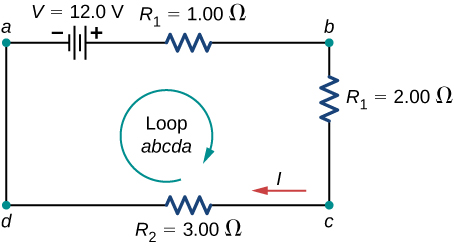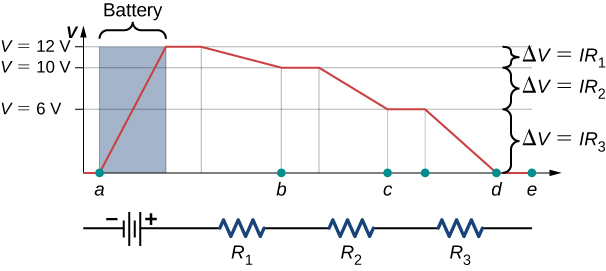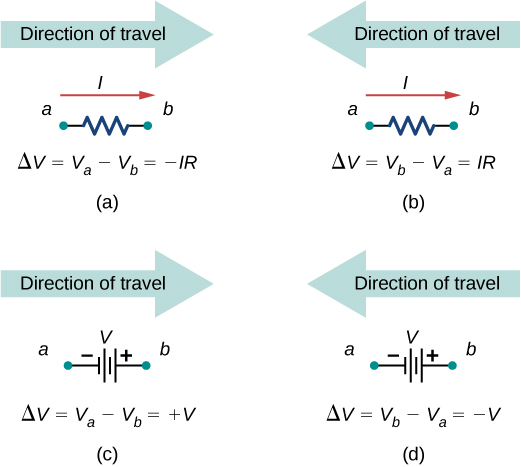10.3 Kirchhoff's rules  (Page 2/10)

 Page 2 / 10A simple loop with no junctions. Kirchhoff’s loop rule states that the algebraic sum of the voltage differences is equal to zero.

The circuit consists of a voltage source and three external load resistors. The labels a , b , c , and d serve as references, and have no other significance. The usefulness of these labels will become apparent soon. The loop is designated as Loop abcda , and the labels help keep track of the voltage differences as we travel around the circuit. Start at point a and travel to point b . The voltage of the voltage source is added to the equation and the potential drop of the resistor ${R}_{1}$ is subtracted. From point b to c , the potential drop across ${R}_{2}$ is subtracted. From c to d , the potential drop across ${R}_{3}$ is subtracted. From points d to a , nothing is done because there are no components.

[link] shows a graph of the voltage as we travel around the loop. Voltage increases as we cross the battery, whereas voltage decreases as we travel across a resistor. The potential drop    , or change in the electric potential, is equal to the current through the resistor times the resistance of the resistor. Since the wires have negligible resistance, the voltage remains constant as we cross the wires connecting the components.A voltage graph as we travel around the circuit. The voltage increases as we cross the battery and decreases as we cross each resistor. Since the resistance of the wire is quite small, we assume that the voltage remains constant as we cross the wires connecting the components.

Then Kirchhoff’s loop rule states

$V-I{R}_{1}-I{R}_{2}-I{R}_{3}=0.$

The loop equation can be used to find the current through the loop:

$I=\frac{V}{{R}_{1}+{R}_{2}+{R}_{2}}=\frac{12.00\phantom{\rule{0.2em}{0ex}}\text{V}}{1.00\phantom{\rule{0.2em}{0ex}}\text{Ω}+2.00\phantom{\rule{0.2em}{0ex}}\text{Ω}+3.00\phantom{\rule{0.2em}{0ex}}\text{Ω}}=2.00\phantom{\rule{0.2em}{0ex}}\text{A}.$

This loop could have been analyzed using the previous methods, but we will demonstrate the power of Kirchhoff’s method in the next section.

Applying kirchhoff’s rules

By applying Kirchhoff’s rules, we generate a set of linear equations that allow us to find the unknown values in circuits. These may be currents, voltages, or resistances. Each time a rule is applied, it produces an equation. If there are as many independent equations as unknowns, then the problem can be solved.

Using Kirchhoff’s method of analysis requires several steps, as listed in the following procedure.

Problem-solving strategy: kirchhoff’s rules

1. Label points in the circuit diagram using lowercase letters a , b , c , …. These labels simply help with orientation.
2. Locate the junctions in the circuit. The junctions are points where three or more wires connect. Label each junction with the currents and directions into and out of it. Make sure at least one current points into the junction and at least one current points out of the junction.
3. Choose the loops in the circuit. Every component must be contained in at least one loop, but a component may be contained in more than one loop.
4. Apply the junction rule. Again, some junctions should not be included in the analysis. You need only use enough nodes to include every current.
5. Apply the loop rule. Use the map in [link] .Each of these resistors and voltage sources is traversed from a to b . (a) When moving across a resistor in the same direction as the current flow, subtract the potential drop. (b) When moving across a resistor in the opposite direction as the current flow, add the potential drop. (c) When moving across a voltage source from the negative terminal to the positive terminal, add the potential drop. (d) When moving across a voltage source from the positive terminal to the negative terminal, subtract the potential drop.

in the 2nd example, for chapter 8.2 on page 3/3, I don't understand where the value 48uC comes from, I just couldn't get that value in my calculator.
what is electromagnetic force. do electric and magnetic force happen differently
yes
yes
Pranay
why
Godson
how?
Godson
derived the electric potential due to disk of charge
how can we derived potential electric due to the disk
aron
how can you derived electric potential of a disk
aron
how can you derived electric potential due to disk
aron
where is response?
aron
what is difference between heat and temperature?
temperature is the measure of degree of hotness or coldness. on the other hand, heat is the form of energy, which causes temperature. So we can safely say, heat is the reason and temperature is its consequence.
SABYASACHI
how many liquid metals do we have
do we have gasses as metals
Jeffery
who knows should please tell us
yes...gallium & cesium
Idris
Hg is liquid. No metal gasses at standard temp and pressure
Shane
I don't ever understand any of this formulae
which formula
How to determine a temperature scale
what is the formula for absolute error
Nyro
define kelvin planck statement
using p-v diagram, explain what takes place during each four processes of a carnot cycle
Masia
using p-v diagram, explain what takes place during each four processes of a carnot cycle
Masia
state zeroths law of thermodynamics
The zeroth law of thermodynamics states that if twothermodynamic systems are each in thermal equilibrium with a third one, then they are in thermal equilibrium with each other
Seza
zeroth law of thermodynamic state that when a body A and B are in thermal equilibrium to body C ,a state is attained when body A,B and C are in thermal equilibrium.
akanbi
sorry guys I got no any idea on the law can someone help me please?
SNAHR
hi
Muhsin
The zeroth law of thermodynamics states that if two thermodynamic systems are each in thermal equilibrium with a third one, then they are in thermal equilibrium with each other. Accordingly, thermal equilibrium between systems is a transitive relation. Two systems are said to be in the relation of
Muhsin
I can help u Snahr as possible
Vinayaka
it simply states that, if two bodies are separately in thermal equilibrium with a third body, then those two bodies will also be in thermal equilibrium with each other
Vinayaka
What mass of steam of 100 degree celcius must be mixed with 150g of ice at 0 degree celcius, in a thermally insulated container, to produce liquid water at 50 degree celcius
sorry I dont know
Bamidele
thank you
Emmanuel
What is the pressure?
SHREESH
To convert 0°C ice to 0°c water. Q=M*s=150g*334J/g=50100 J.......... Now 0° water to 50° water... Q=M*s*dt=150g*4.186J/g*50= 31395 J....... Which adds upto 81495 J..... This is amount of heat the steam has to carry. 81495= M *s=M*2230J/g..therefore.....M=36.54g of steam
SHREESH
This is at 1 atm
SHREESH
If there is change in pressure u can refer to the steam table ....
SHREESH
instrument for measuring highest temperature of a body is?
Thermometer
Umar
how does beryllium decay occur
Photon?
Umar
state the first law of thermodynamics
Its state that "energy can neither be created nor destroyed but can be transformed from one form to another. "
Ayodamola
what about the other laws can anyone here help with it please
Sandy
The second law of thermodynamics states that the entropy of any isolated system always increases. The third law of thermodynamics states that the entropy of a system approaches a constant value as the temperature approaches absolute zero.
sahil
The first law is very simple to understand by its equation. The law states that "total energy in thermodynamic sytem is always constant" i.e d¶=du+dw where d¶=total heat du=internal energy dw=workdone... PLEASE REFER TO THE BOOKS FOR MORE UNDERSTANDING OF THE CONCEPT.
Elia
what is distance.?
what is physics?
Ali
Physics is a scientific phenomenon that deals with matter and its properties
Ayodamola
physics is the study of nature and science
John
Chater1to7
min
Physics is branch of science which deals with the study of matters in relation with energy.
Elia
What is differential form of Gauss's law?By